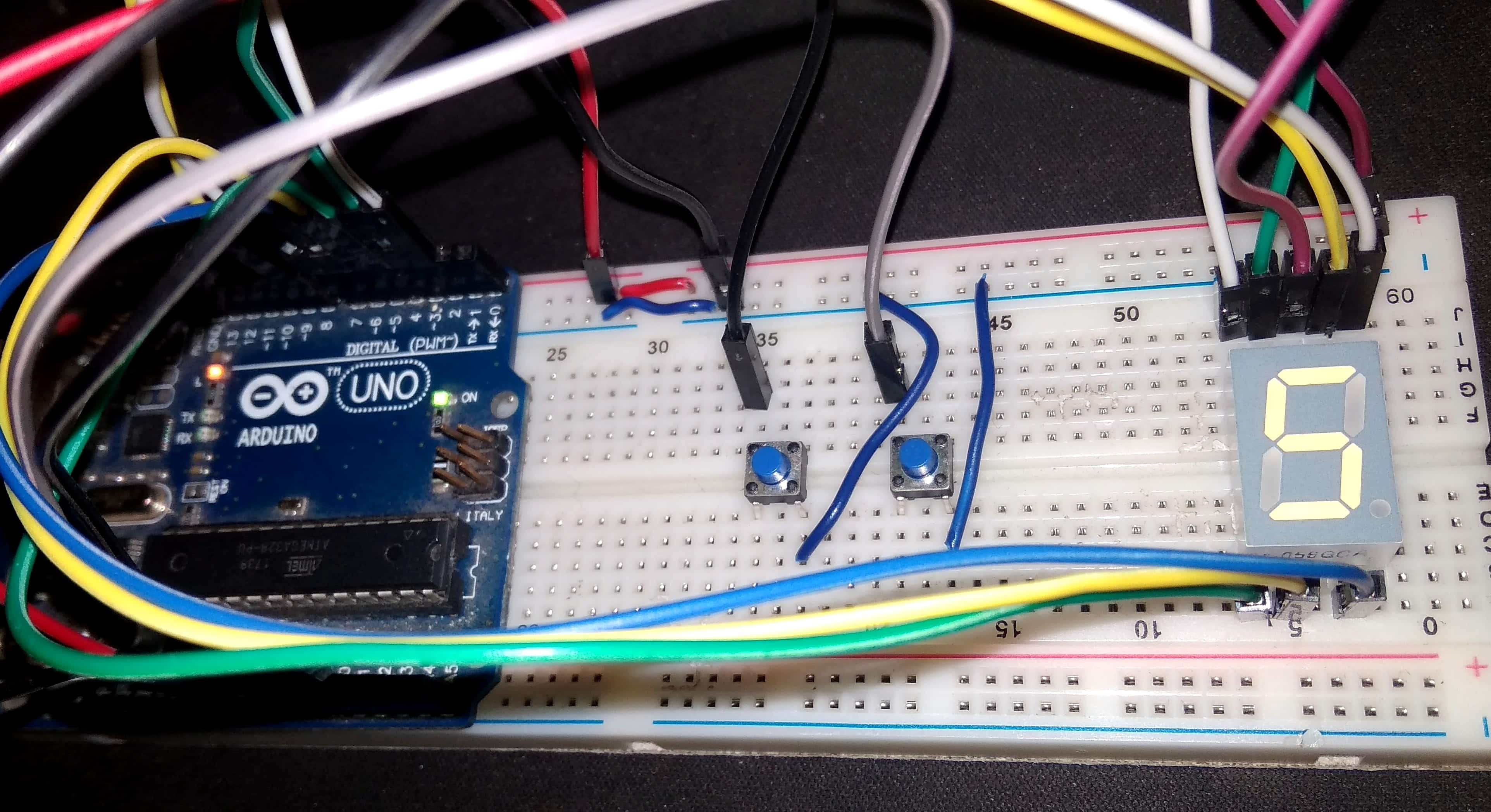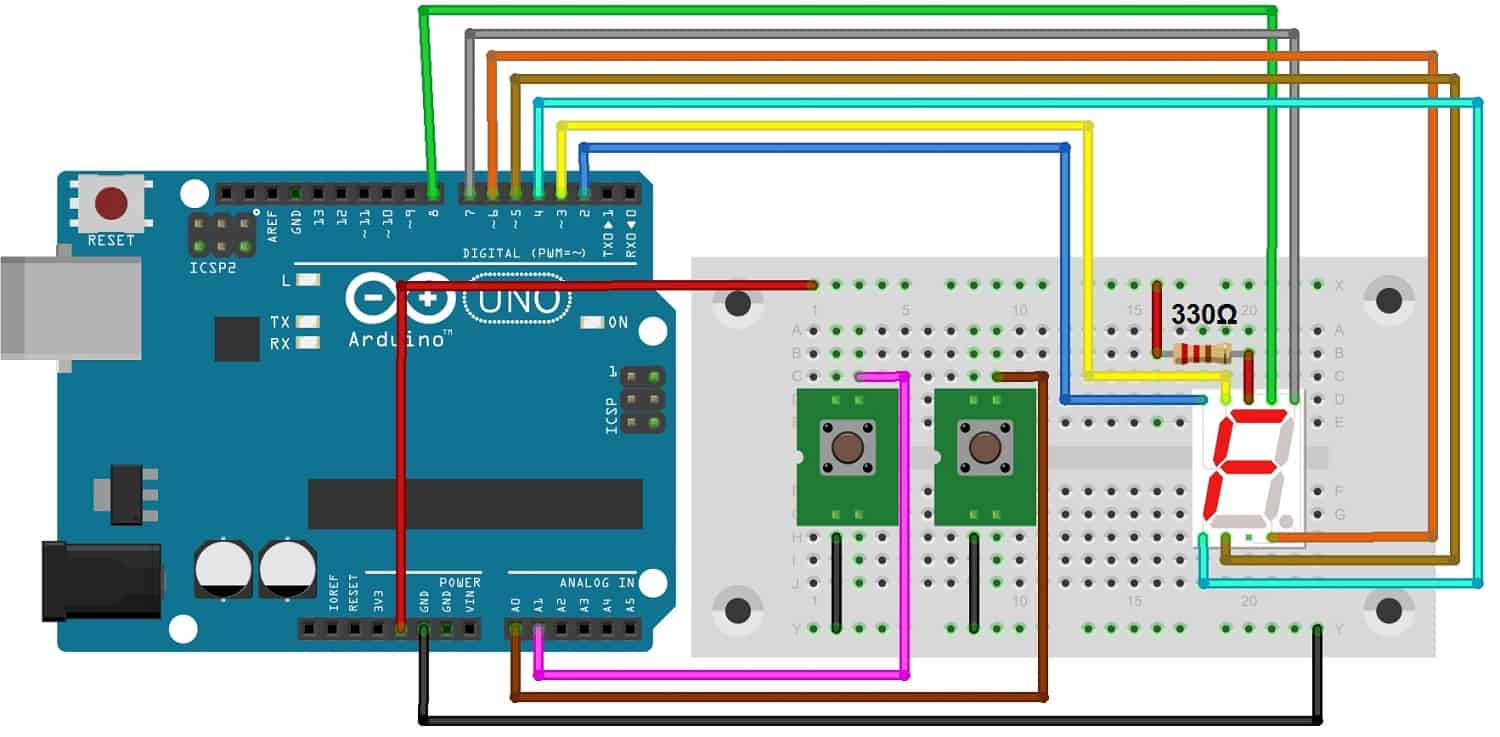Digital Dice using Arduino & 7 Segment Display – Electronic Dice(Last Updated On: February 25, 2019)

Digital Dice using Arduino & 7 Segment Display:

In this project we have interfaced 7 segment display with Arduino to design an electronic dice. In fact its a simple Digital Dice using Arduino & 7 Segment Display. This dice can be used while playing Ludo or Ladder game.

Here you don’t need to throw a dice to get a number. Instead you need to press a button to get a random number between 1 to 6.

Components Required:

```1. Arduino UNO
2. Seven Segment Display (Common Anode)
3. Push buttons - 2
4. Connecting wires
6. 330 ohm resistor```

Circuit Diagram & Connections:

To design Digital Dice using Arduino & 7 Segment Display make the following connections as shown in the circuit diagram below.Connect the push buttons to Analog pins A0 & A1 of Arduino.

Connect the common terminal of 7 segment display to 5V via 330 ohm resistor. Connect the terminal a,b,c,d,e,f,g,h of 7 segment display to digital pin 8,7,6,5,4,3,2 of Arduino respectively. The 7 segment dipaly that we have used here is common anode 7 segment display which has 2 common terminals that need 5V power supply for operations.Source Code/Program:

Here is a code for Electronic Dice using Arduino.

```char digit={0x02, 0x79, 0x24, 0x30, 0x19, 0x12};
int pin={8,7,6,5,4,3,2};

#define rst A1
#define dice A0

void setup()
{
for(int i=0;i<7;i++)
pinMode(pin[i], OUTPUT);
pinMode(dice, INPUT);
pinMode(rst, INPUT);
digitalWrite(dice, 1);
digitalWrite(rst, 1);
int temp=0x40;
for(int i=0;i<7;i++)
{
int x=temp&0x01;
digitalWrite(pin[i], x);
temp=temp>>1;
}
delay(1000);
}

void loop()
{
int temp=rand();
{
int k=temp%6;
temp=digit[k];
wait();
for(int i=0;i<7;i++)
{
int x=temp&0x01;
digitalWrite(pin[i], x);
temp=temp>>1;
}
delay(200);
}

{
temp=0x40;
for(int i=0;i<7;i++)
{
int x=temp&0x01;
digitalWrite(pin[i], x);
temp=temp>>1;
}
}
}

void wait()
{
for(int m=0;m<10;m++)
{
for(int k=0;k<6;k++)
{
int ch=digit[k];
for(int l=0;l<7;l++)
{
char y=ch&0x01;
digitalWrite(pin[l], y);
ch=ch>>1;
}
delay(40);
}
}
}```

Code Explanation:

First of all we selected digital pin 8,7,6,5,4,3,2 for seven segment display. Then we created the array of 6 digits as 0x02, 0x79, 0x24, 0x30, 0x19, 0x12 to display 1,2,3,4,5,6. Then input and output pins are defined. By default the command will display 0 when code is uploaded. The rand() function is used to get a random number.

When we press the dice button program first map this random number and then send number to 7 segment display by using bit wise operator. the reset button will restore the value to 0.

Recommended For You